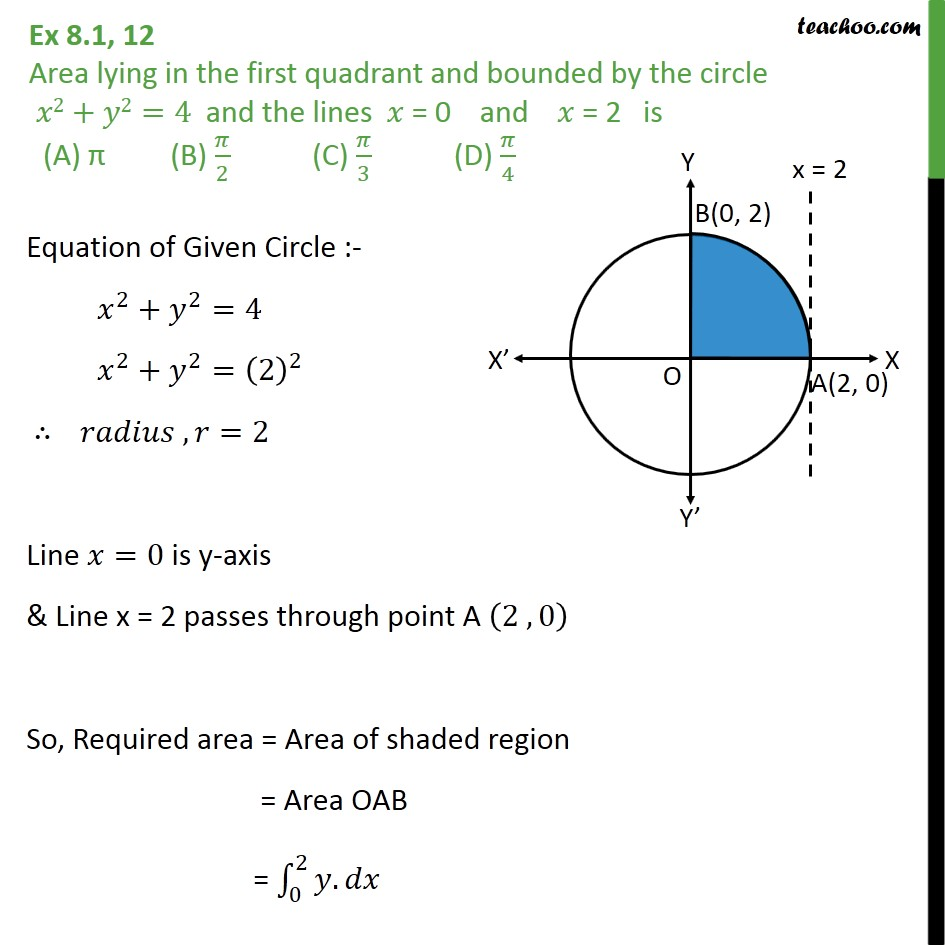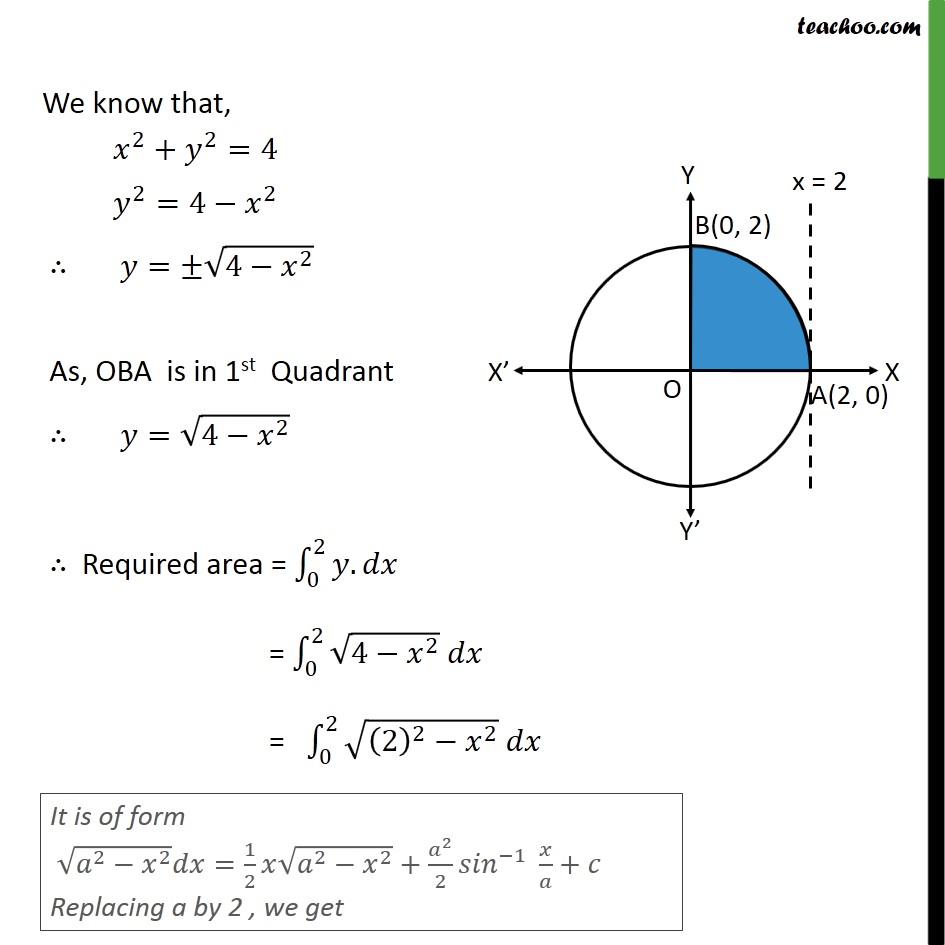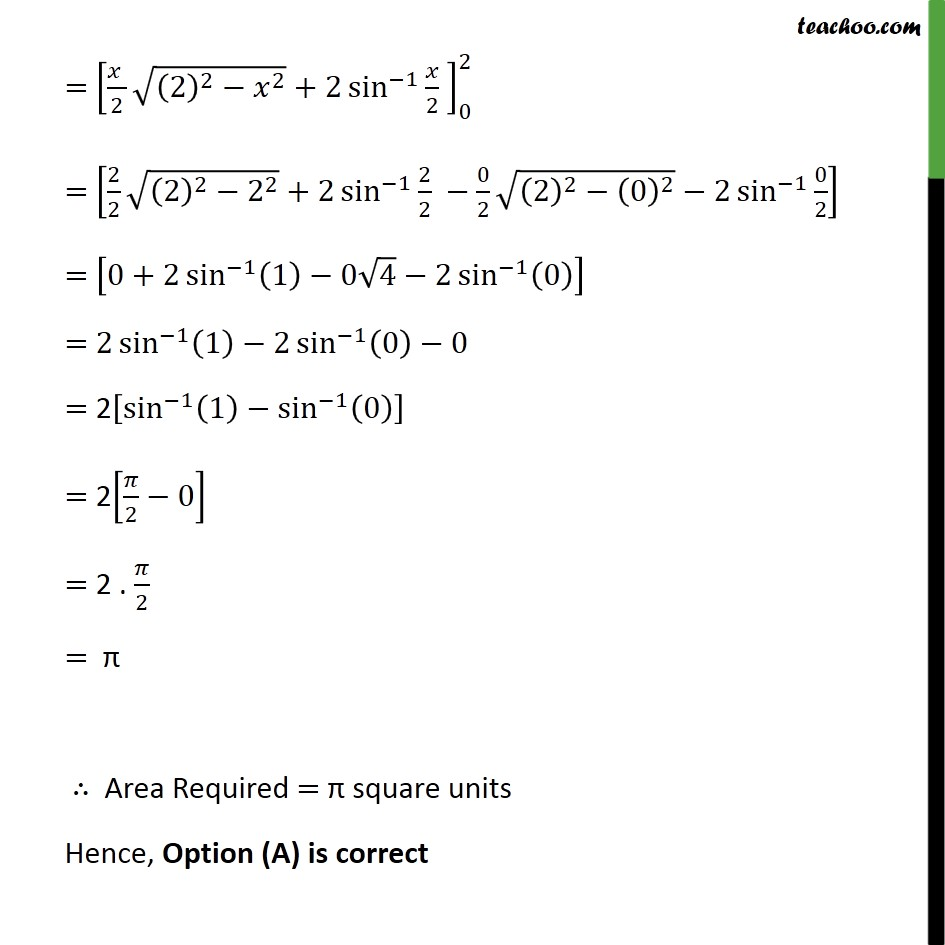Area bounded by curve and horizontal or vertical line

Chapter 8 Class 12 Application of Integrals
Concept wiseLearn in your speed, with individual attention - Teachoo Maths 1-on-1 Class

### Transcript

Ex 8.1, 12 Area lying in the first quadrant and bounded by the circle 𝑥2+𝑦2=4 and the lines 𝑥 = 0 and 𝑥 = 2 is (A) π (B) 𝜋﷮2﷯ (C) 𝜋﷮3﷯ (D) 𝜋﷮4﷯ Equation of Given Circle :- 𝑥﷮2﷯+ 𝑦﷮2﷯=4 𝑥﷮2﷯+ 𝑦﷮2﷯= 2﷯﷮2﷯ ∴ 𝑟𝑎𝑑𝑖𝑢𝑠 , 𝑟=2 Line 𝑥=0 is y-axis & Line x = 2 passes through point A 2 , 0﷯ So, Required area = Area of shaded region = Area OAB = 0﷮2﷮𝑦.𝑑𝑥﷯ We know that, 𝑥﷮2﷯+ 𝑦﷮2﷯=4 𝑦﷮2﷯=4− 𝑥﷮2﷯ ∴ 𝑦=± ﷮4− 𝑥﷮2﷯﷯ As, OBA is in 1st Quadrant ∴ 𝑦= ﷮4− 𝑥﷮2﷯﷯ ∴ Required area = 0﷮2﷮𝑦.𝑑𝑥﷯ = 0﷮2﷮ ﷮4− 𝑥﷮2﷯﷯ 𝑑𝑥﷯ = 0﷮2﷮ ﷮ 2﷯﷮2﷯− 𝑥﷮2﷯﷯ 𝑑𝑥﷯ = 𝑥 ﷮2﷯ ﷮ 2﷯﷮2﷯− 𝑥﷮2﷯﷯+2 sin﷮−1﷯﷮ 𝑥﷮2﷯ ﷯﷯﷮0﷮2﷯ = 2﷮2﷯ ﷮ 2﷯﷮2﷯− 2﷮2﷯﷯+2 sin﷮−1﷯﷮ 2﷮2﷯ ﷯− 0﷮2﷯ ﷮ 2﷯﷮2﷯− 0﷯﷮2﷯﷯−2 sin﷮−1﷯﷮ 0﷮2﷯﷯﷯ = 0+2 sin﷮−1﷯﷮ 1﷯−0 ﷮4﷯−2 sin﷮−1﷯﷮ 0﷯﷯﷯﷯ = 2 sin﷮−1﷯﷮ 1﷯−2 sin﷮−1﷯﷮ 0﷯﷯﷯−0 = 2 sin﷮−1﷯﷮ 1﷯− sin﷮−1﷯﷮ 0﷯﷯﷯﷯ = 2 𝜋﷮2﷯−0﷯ = 2 . 𝜋﷮2﷯ = π ∴ Area Required = π square units Hence, Option (A) is correct